Enable Dark Mode!By: Shabna P

# Asset Management Documentation in Odoo 14

## Functional Odoo 14 Accounting

Asset management is a systematic process to organize, monitor, and sell the assets of an organization in a cost-effective way. By monitoring the assets and their current working conditions, will help the business to understand the loss/gain, equipment failures, etc.

Odoo 14 also provides the feature of asset model and asset creation to manage the assets in a company. The asset model is a feature to manage the asset entries for an asset. The depreciation for the asset can be set for a particular duration. A single asset model can be used for creating multiple assets

Asset Model Creation

To create an asset model, Go to Accounting module -> Configuration -> Management -> Asset Model -> Create

Create an asset model by adding details as required in the form.Asset Model Name: Name described for the asset model

Depreciation Method: Assent depreciation can be calculated as:

1.  Straight line: This will calculate the depreciation linearly, ie equally split for each year

2.  Degressive: For this type of depreciation, the actual amount will multiply with a degressive factor, which is the depreciable amount.

3.  Accelerated Degressive: It is a combination of straight lines and degressive depreciation. From the start, the depreciation is calculated by multiplying with a factor, as the same as degressive and when it reaches the linear value, it will follow the straight-line method.

Duration: No. of depreciation needed for the asset

Prorata Temporis: This is a feature to determine from which date the depreciation needs to calculate. If checked, the first date of the depreciation starts from the asset creation date (Purchase date).

Accounting:

1.  Fixed Asset Account: The account which was used to document the purchasing of the asset at its original cost

2.  Depreciation Account: Account used to record the depreciation entries

3.  Expense account: Accounts used to report the expense portion of the asset.

4.  Journal

One can mention the analytical account and analytical tag if needed. Thus the asset model is created.

Now go to the chart of accounts and assign the asset model to the asset account, Accounting Module -> Configuration -> Accounting -> Chart of Accounts. Edit fixed asset account.Under Asset options, 'Automate Asset' chosen as Create and Validate and created asset model is added on the field. Then, save changes.

Purchase Asset

Now let see how the purchased product can be converted to an asset using the asset account and asset model. Create a purchase invoice from the accounting module and add the asset account.Add the label for the vendor bill, then add an account, quantity, and price. Now confirm the bill.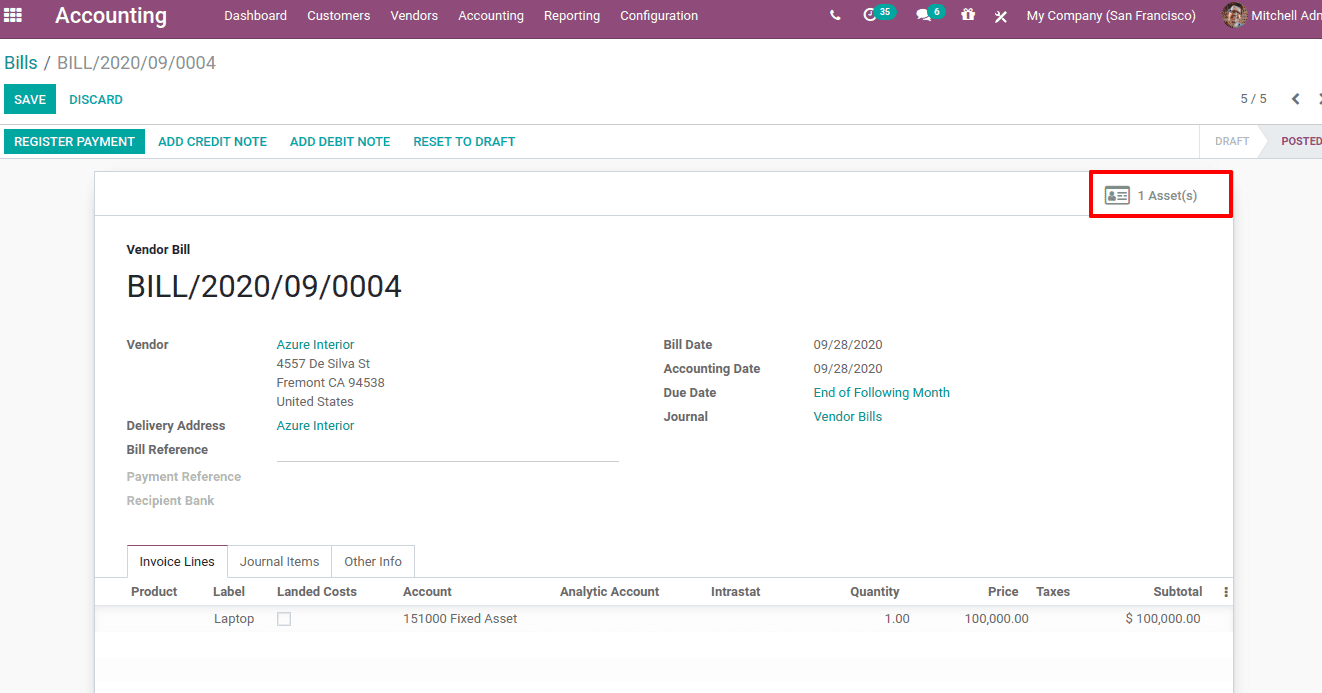On confirming a smart button 'Asset (s)' will appear. Details on assets can be viewed in the asset tab.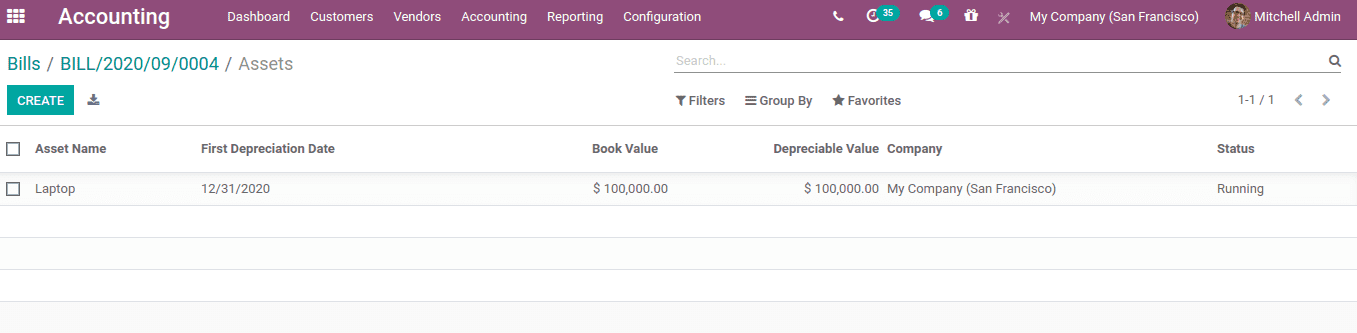Thus the asset is created, whose first depreciation date, value, and status can be seen here. Click on the asset, which will show the depreciation entries for each year.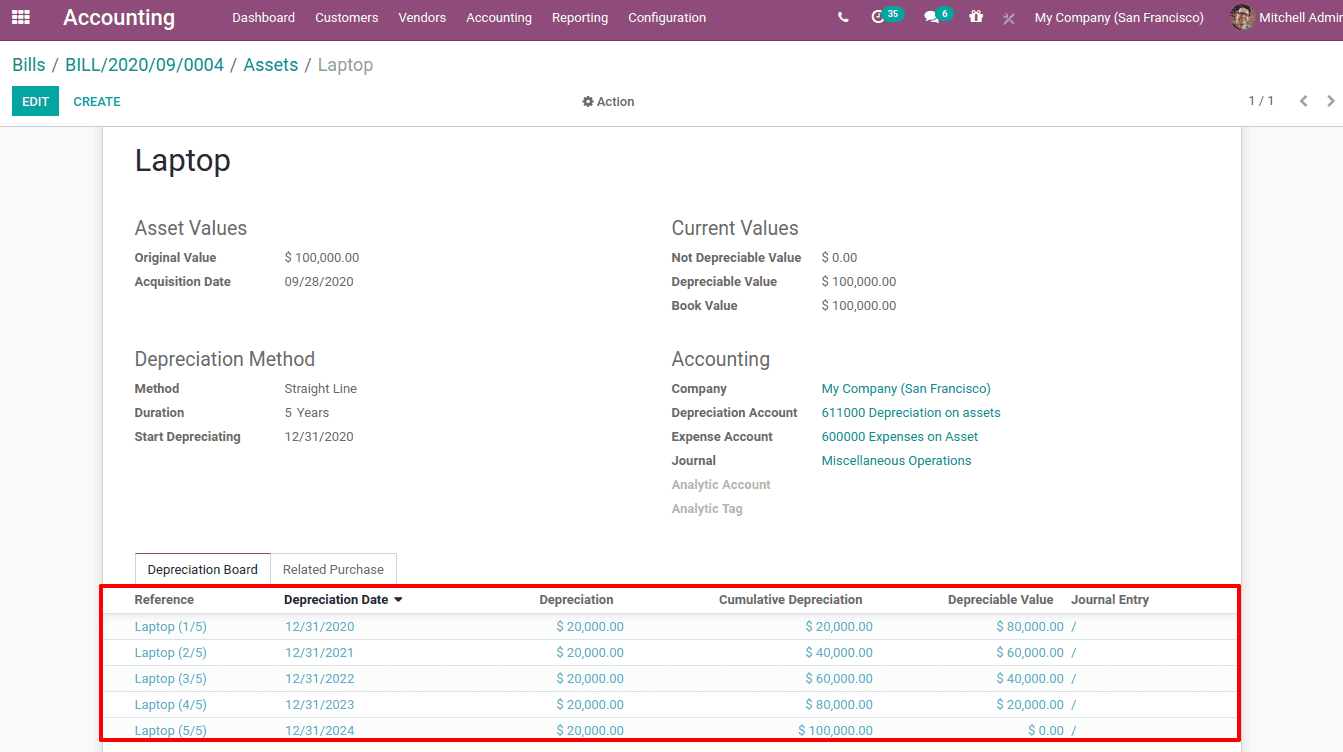Here you can see the original value of the asset, acquisition date, and all the calculation of depreciation for the 5 years can be viewed under the 'Depreciation Board' tab.Here you have a smart tab 'Posted Entries'. It will display the details of all posted depreciation.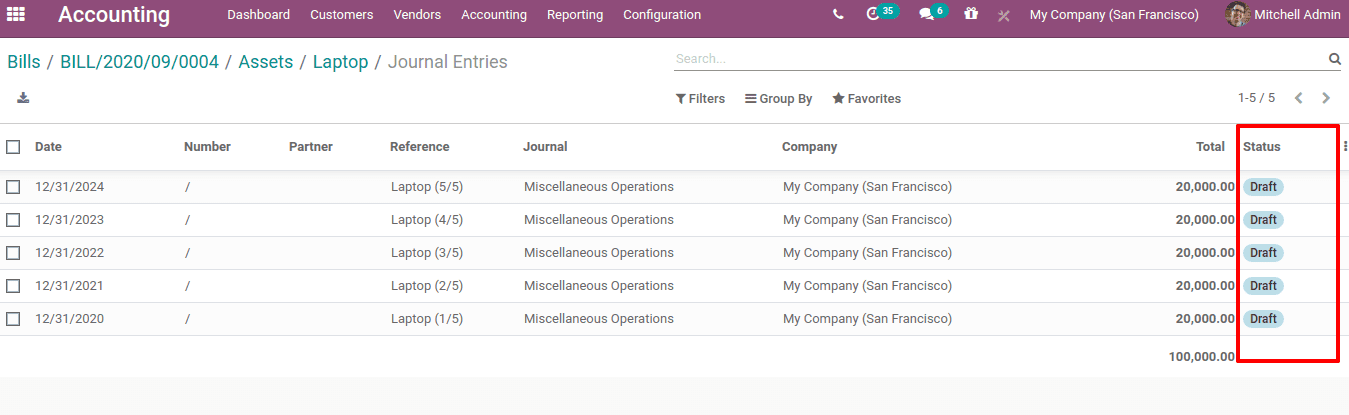For each depreciation, there is a depreciation date. The depreciation entries are validated and posted on the corresponding dates. Right Now, the status is in Draft.Once the entry is posted, status changes to post. In this case, at the top, a message is displayed "This move is configured to be posted automatically at the accounting date: 12/31/2020". Thus the journal entries are recorded in similar accounts on that particular accounting date. In the other info tab, you can see the checkbox 'post automatically' is ticked.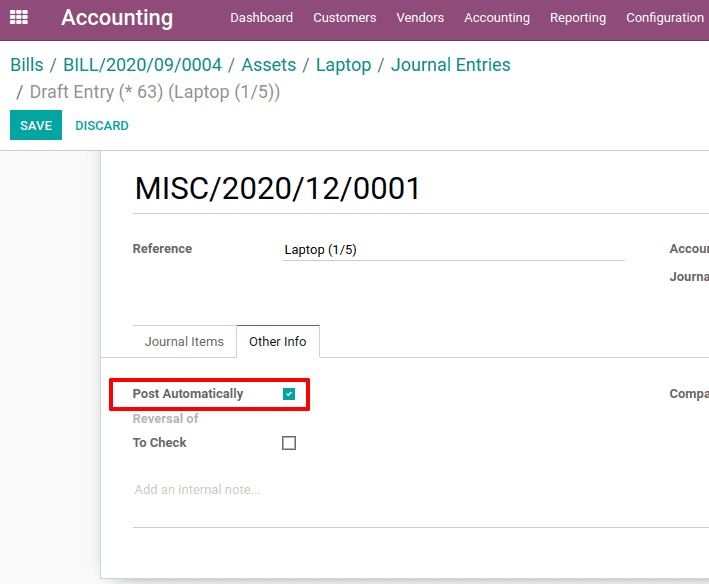It means the accounting entry is automatically posted on that particular accounting date.

We can manually post this entry, by un-ticking the option 'post automatically'. Now post the entry. So the assets will be as shown below.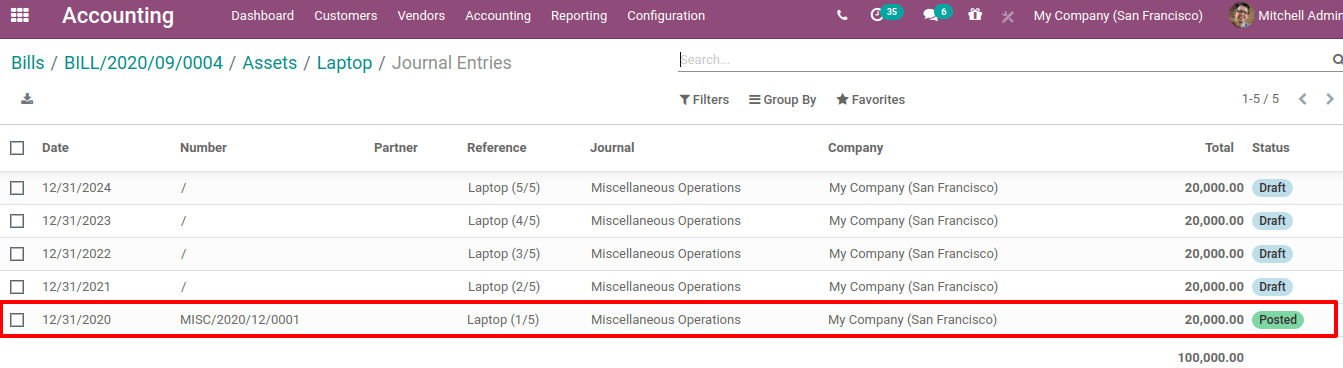The posted entries will be reflected in the profit and loss account.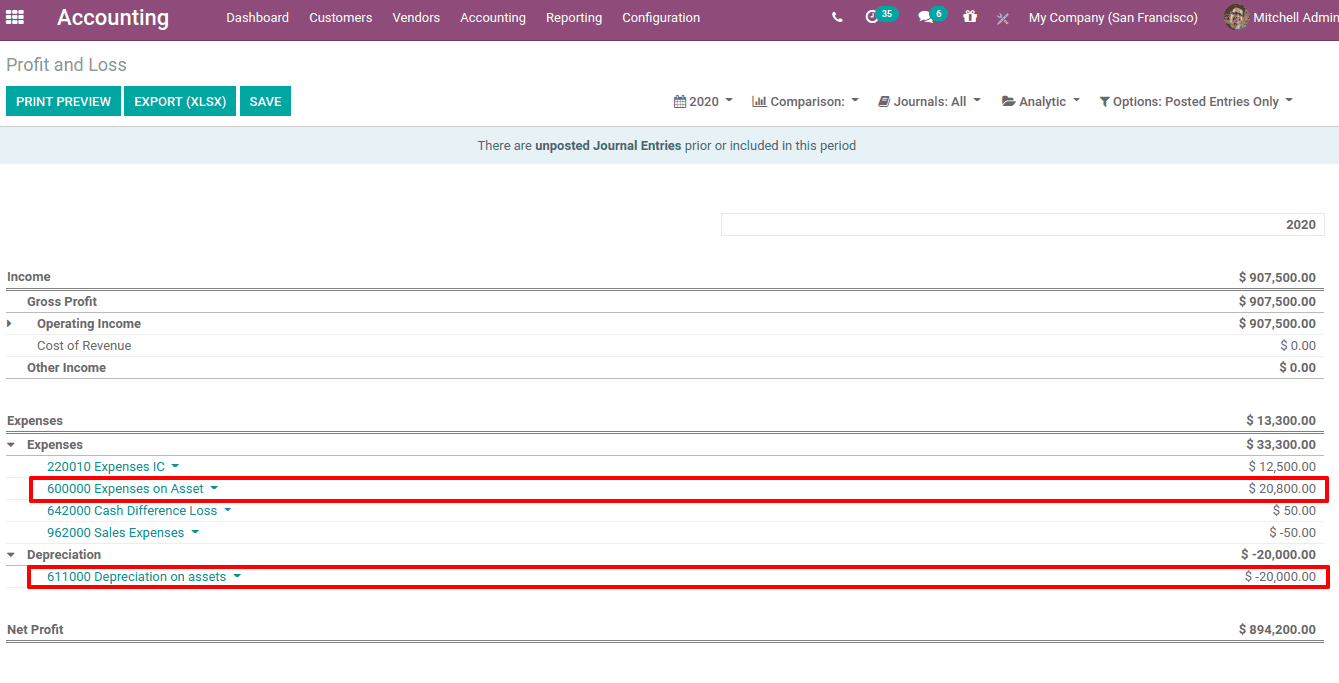One can save the asset model using 'SAVE MODEL' or pause or modify depreciation using 'PAUSE DEPRECIATION' and 'MODIFY DEPRECIATION button respectively.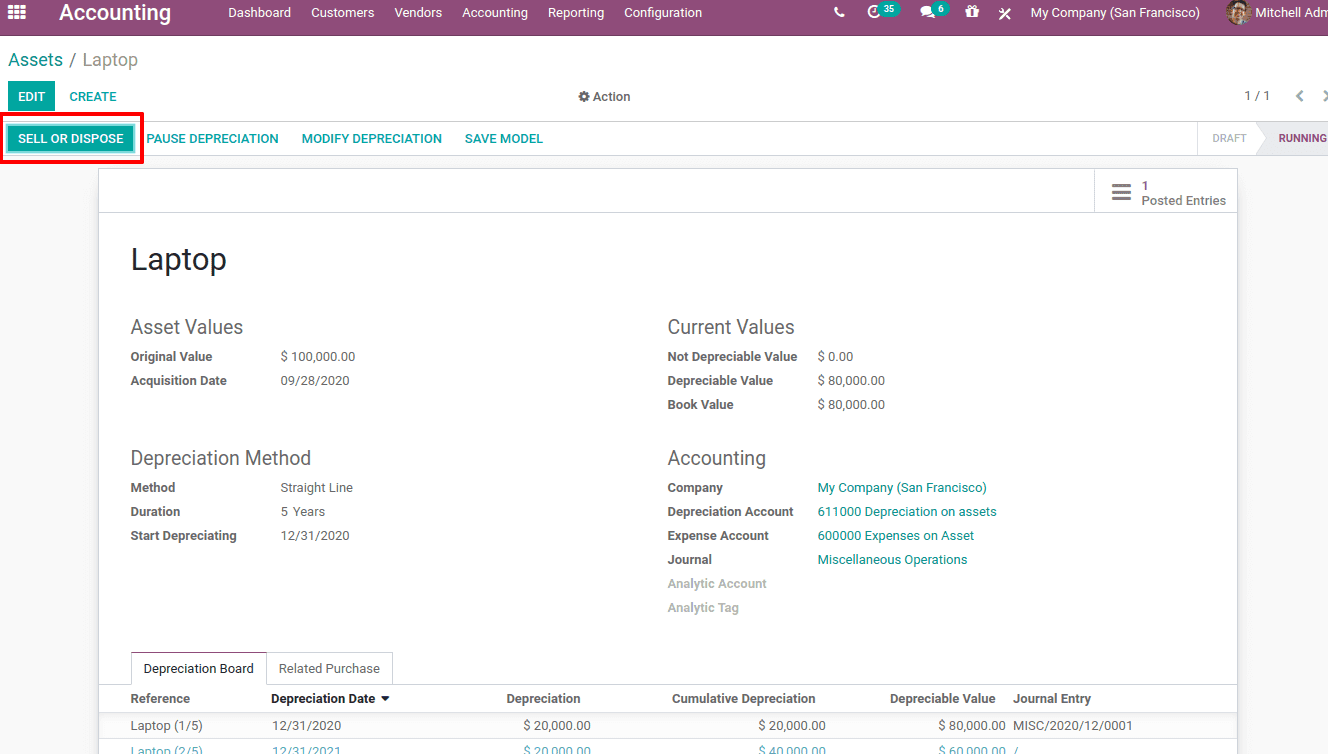Also, the asset can be 'sell' or 'dispose' at the end. If the action is 'sell', mention the customer invoice and Loss / Gain account.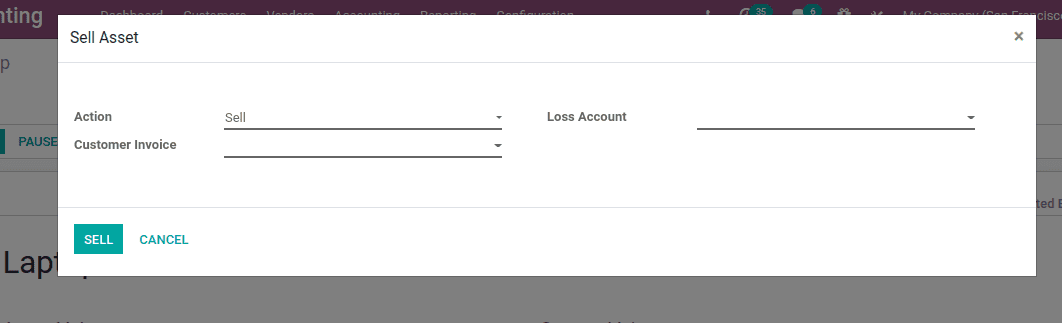For an action 'Dispose', mention the Loss/Gain account.

If you need any assistance in odoo, we are online, please chat with us.

### Recent Posts

#### How to Categorize Products Using Odoo 17 Purchase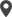Calicut

Cybrosys Technologies Pvt. Ltd.
Neospace, Kinfra Techno Park
Kakkancherry, Calicut
Kerala, India - 673635London

Cybrosys Limited
Alpha House,
100 Borough High Street, London,
SE1 1LB, United KingdomKochi

Cybrosys Technologies Pvt. Ltd.
1st Floor, Thapasya Building,
Kochi, India - 682030.Bangalore

Cybrosys Techno Solutions
The Estate, 8th Floor,